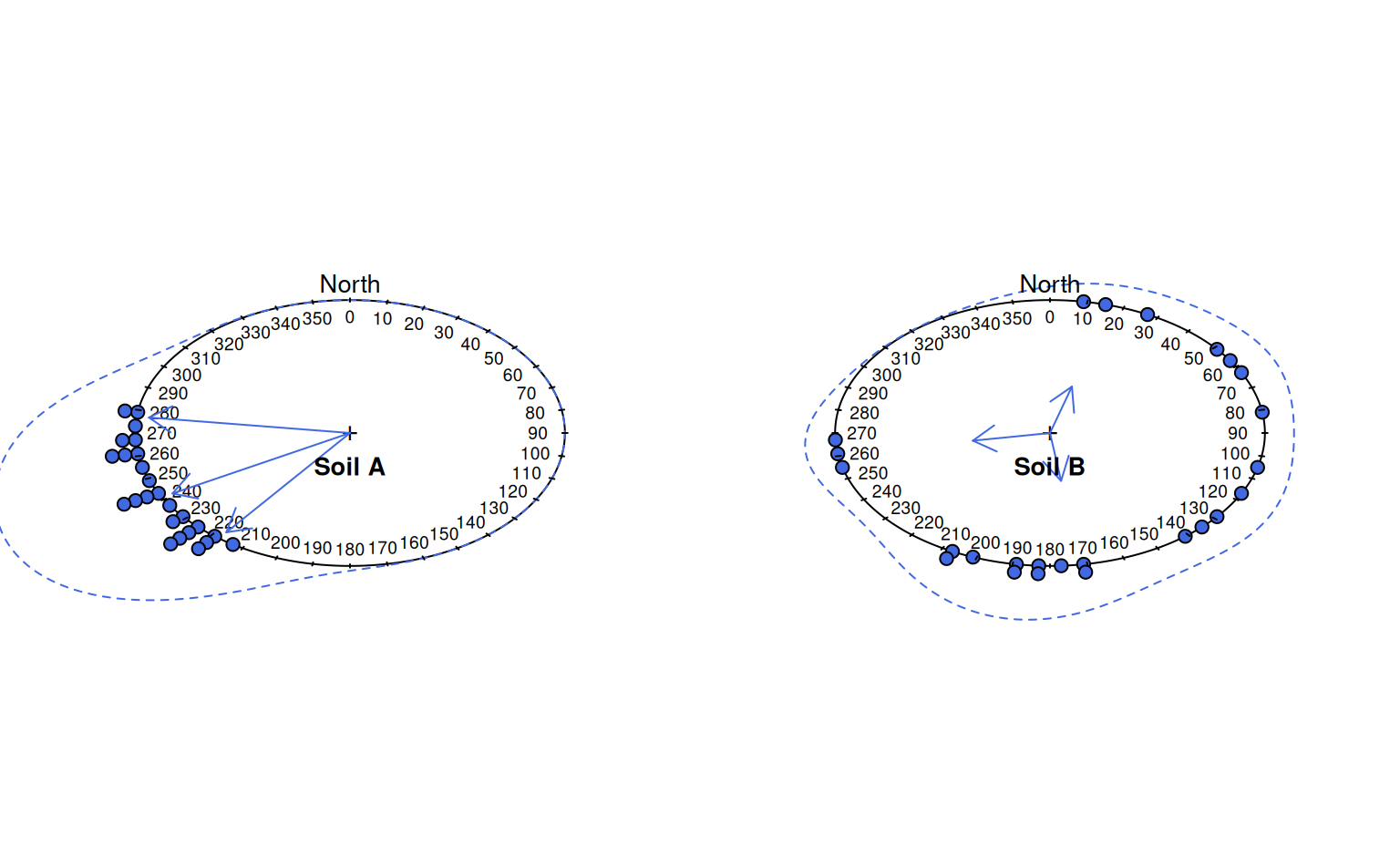Plot a graphical summary of multiple aspect measurements on a circular diagram.

aspect.plot(
p,
q = c(0.05, 0.5, 0.95),
p.bins = 60,
p.bw = 30,
stack = TRUE,
p.axis = seq(0, 350, by = 10),
plot.title = NULL,
line.col = "RoyalBlue",
line.lwd = 1,
line.lty = 2,
arrow.col = line.col,
arrow.lwd = 1,
arrow.lty = 1,
arrow.length = 0.15,
...
)

## Arguments

p

a vector of aspect angles in degrees, measured clock-wise from North

q

a vector of desired quantiles

p.bins

number of bins to use for circular histogram

p.bw

bandwidth used for circular density estimation

stack

logical, should the individual points be stacked into p.bins number of bins and plotted

p.axis

a sequence of integers (degrees) describing the circular axis

plot.title

an informative title

line.col

density line color

line.lwd

density line width

line.lty

density line line style

arrow.col

arrow color

arrow.lwd

arrow line width

arrow.lty

arrow line style

arrow.length

...

further arguments passed to circular::plot.circular

## Value

invisibly returns circular stats

## Details

Spread and central tendency are depicted with a combination of circular histogram and kernel density estimate. The circular mean, and relative confidence in that mean are depicted with an arrow: longer arrow lengths correspond to greater confidence in the mean.

## Note

Manual adjustment of p.bw may be required in order to get an optimal circular density plot. This function requires the package circular, version 0.4-7 or later.

D.E. Beaudette

## Examples

# simulate some data
p.narrow <- runif(n=25, min=215, max=280)
p.wide <- runif(n=25, min=0, max=270)

# set figure margins to 0, 2-column plot
par(mar = c(0,0,0,0), mfcol = c(1,2))

# plot, save circular stats
x <- aspect.plot(p.narrow, p.bw=10, plot.title='Soil A', pch=21, col='black', bg='RoyalBlue')
#>
#>        Rayleigh Test of Uniformity
#>        General Unimodal Alternative
#>
#> Test Statistic:  0.9489
#> P-value:  0
#>
y <- aspect.plot(p.wide, p.bw=10, plot.title='Soil B', pch=21, col='black', bg='RoyalBlue')#>
#>        Rayleigh Test of Uniformity
#>        General Unimodal Alternative
#>
#> Test Statistic:  0.4067
#> P-value:  0.0146
#>

# reset output device options
par(op)

x
#> Circular Data:
#> Type = angles
#> Units = degrees
#> Template = geographics
#> Modulo = 2pi
#> Zero = 1.570796
#> Rotation = clock
#>       5%      50%      95%
#> 275.3298 245.3053 223.8753